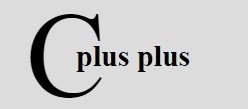# C++ Type Conversion & Type Casting

« Previous Tutorial Next Tutorial »

## Type Conversion

The process of converting one predefined type into another is called as type conversion. When constants and variables of different types are mixed in an expression, they are converted to the same type. When variables of one type are mixed with variables of another type, a type conversion will occur. C++ facilitates the type conversion into the following two forms :

• Implicit Type Conversion
• Explicit Type Conversion

## Implicit Type Conversion

An implicit type conversion is a conversion performed by the compiler without programmer's intervention. An implicit conversion is applied generally whenever differing data types are intermixed in an expression (mixed mode expression), so as not to lose information. The value of the right side (expression side) of the assignment is converted to the type of the left side (target variable).

Therefore, the types of right side and left side of an assignment should be compatible so that type conversion can take place. The compatible data types are mathematical data types i.e., char, int ,float, double. For example, the following statement :

`ch = x ;     (where ch is char and x is int)`

Converts the value of x i.e., integer to bit into ch, a character. Assuming that word size is 16-bit (2 bytes), the left higher order bits of the integer variable x are looped off, leaving ch with lower 8-bits. Say, if x was having value 1417 (whose binary equivalent is 0000010110001001) the ch will have lower 8-bits i.e., 10001001 resulting in loss of information.

The C++ compiler converts all operands upto the type of the largest operand, which is called type promotion. This is done operation by operation, as described in the following type conversion algorithm :

• If either operand is of type long double, the other is converted to long double.
• Otherwise, if either operand is of type double, the other is converted to double.
• Otherwise, if either operand is float, the other is converted to float.
• Otherwise, the integral promotions are performed on both operands. The process of integral promotion is described below :
A char, a short int, enumerator or an int (in both their signed and unsigned varieties) may be used as an integer type. If an int can represent all the values of the original type, the value is converted to int ; otherwise it is converted to unsigned int. This process is called integral promotion.
• Then, if either operand is unsigned long, the other is converted to unsigned long.
• Otherwise, if one operand is a long int and the other unsigned int, then if a long int can represent all the values of an unsigned int, the unsigned int is converted to long int; otherwise both operands are converted to unsigned long int.
• Otherwise, if either operand is long, the other is converted to long.
• Otherwise, if either operand is unsigned, the other is converted to unsigned.
• Otherwise, both operands are int.

When converting from integers to characters and long integers to integers, the appropriate amount of high-orders bits (depending upon target type's size) will be removed. In many environments, this means that 8 bits will be lost when going from an integer to a character and 16 bits will be lost when going from a long integer to an integer.

The following table summarizes the assignment type conversions :

Common Type Conversion (assuming a 16-bit word)
Target Type Expression Type Possible Info Loss
signed char char if value > 127, target is negative
char short int High-order 8 bits
char int High-order 8 bits
char long int High-order 8 bits
int long int High-order 16 bits
int float Fractional part and possibly more
float double Precision, result rounded
double long double Precision, result rounded

Remember - When conversion takes place from smaller type to longer type e.g., from int to float or a float to a double, there is no loss of information. Neither does it add any precision or accuracy. These kinds of conversions only change the form in which the value is represented.

## Explicit Type Conversion - Type Casting

The explicit conversion of an operand to a specific type is called type casting. An explicit type conversion is user-defined that forces an expression to be of specific type.This is the general form to perform type casting in C++

`(type) expression`

where type is a valid C++ data type to which the conversion is to be done. For example, to make sure that expression (x + y/2) evaluates to type float, write it as :

`(float) (x + y/2)`

As you can see from the above statement, the resultant types of the right side can be converted explicitly using type casting operator (). For example, the resultant type of the following expression side is double.

```int i, j;
float f, result;
double d;
result = (i/j) + (f/d) - (f+i);```

If you want to convert the type of expression side to float explicitly, it can be done as shown below:

`result = (float) ( (i/j) + (f/d) - (f+i));`

Casts are often considered as operators. As an operator, a cast is unary and has the same precedence as any other unary operator. Here is an example

```/* C++ Type Conversion and Type Casting */

#include<iostream.h>
#include<conio.h>
void main()
{
clrscr();
float res;
float f1=15.5, f2=2;
res = (int)f1/(int)f2;
cout<<res<<endl;
res = (int)(f1/f2);
cout<<res<<endl;
res = f1/f2;
cout<<res;
getch();
}```

Here is the sample run of the above C++ program:

C++ Online Test

« Previous Tutorial Next Tutorial »